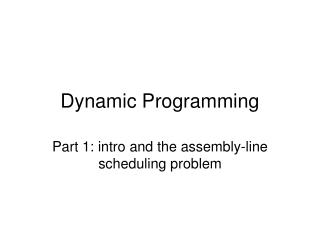DownloadDownload PresentationDynamic Programming

# Dynamic Programming

Download Presentation## Dynamic Programming

- - - - - - - - - - - - - - - - - - - - - - - - - - - E N D - - - - - - - - - - - - - - - - - - - - - - - - - - -
##### Presentation Transcript

1. Dynamic Programming Part 1: intro and the assembly-line scheduling problem

2. What is dynamic programming • Is an algorithm method that solves a problem by combining solutions of subproblems • This sounds similar to divide-and-conquer • However, there’s a difference between the two: • In divide-and-conquer, the subproblems don’t overlap • In dynamic programming, the subproblems overlap • Subproblems share sub-subproblems!

3. Dynamic programming may be good for these problems • Dynamic programming is typically applied to optimization problems • These optimization problems may have many possible solutions • Each solution has a value associated with it • We want a solution with the optimal (min or max) value • We say “a solution,” not “the solution,” because there may be more than 1 solution with the same, best value

4. Sequence in the development of a dynamic programming algorithm • Characterize the structure of an optimal solution • Recursively define the value of an optimal solution • Compute the value of an optimal solution in a bottom-up fashion (smaller subproblems first) • Construct an optimal solution from computed information • Step 4 omitted if only the value of the best solutions is required

5. Assembly line scheduling • 2 assembly lines at an auto assembly plant • Normally they operate independently and concurrently • But when there is a rush job the manager halts the assembly lines and use stations in both assembly lines in an optimal way, to be explained next

6. Step 1: structure of fastest way: What’s the fastest way through station S(1,j)? • First suppose the fastest way through station S(1,j) is though S(1,j-1) • Key observation: the chassis must have taken a fastest way from the starting point through station S(1,j-1) • Why? If there had been a faster way to get through station S(1,j-1), we could have substituted this faster way to yield a faster way through station S(1,j): a contradiction

7. Dynamic optimality or Optimal substructure property An optimal solution to a problem (finding the fastest way through station S(i,j) contians within it an optimal solution to subproblems (finding the fastest way through either S(1,j-1) or s(2,j-1)).

8. Step 2: a recursive solution Let fi[j] = fastest possible time to get a chassis from the starting point through station S(i,j) Ultimate goal: compute fastest time to get a chassis all the way through the factory Denote this time by f* We have…

9. Equations for the first stations on each assembly line

10. Step 3: computing the fastest times

11. Step 4: constructing the fastest way